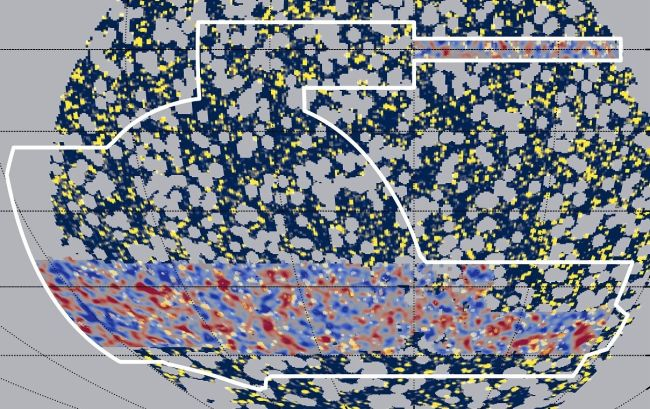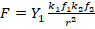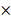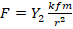# Explanation to why gamma-rays and dark matter density align

https://zenodo.org/record/4062247#.YQh4vkApDIW

The preprint published on May 11 2020

Alexander Z. Yan*, Max Gaofei Yan

300 NE 12th Ave. Apt. 601 Hallandale Beach, FL 33009-4503, USA; *azyan@gwu.edu

ABSTRACT

Recent studies showed that gamma-rays and dark matter density correlate. There is a suggestion that this is because a dark matter particle decays and emits gamma-rays. Based on a new theory of gravity and its equations relating gravity force and photon frequency, we offer a novel explanation for this observation. The new equations show that a higher frequency photon will produce a greater gravitational effect. This makes gamma-rays, the highest frequency EM wave, the strongest producer of dark matter’s gravitational effects. The search for specific frequencies, such as gamma-rays and x-rays, emitted from the decay of a hypothetical dark matter particle is flawed and contradicts observations. If dark matter’s gravitational effects are created by photons, not a particle with rest mass, dark matter observations are satisfied.

INTRODUCTION

While dark matter can be detected through its gravitational effect, dark matter itself has not been directly observed. Because of this, there are many theories on what dark matter is. Many suggest dark matter is some unknown tiny particle with rest mass, but so far, none of these theories have been verified. A recent research paper  seems to build support for a tiny particle theory by finding a correlation between untriggered gamma-ray bursts and a dark matter density map. Other research tried to find dark matter decay by looking for x-rays [2, 3]. These searches for EM waves have brought more questions than answers on the nature of dark matter.

This paper discussed the relationship between dark matter and photons based on the gamma-ray observations. As an extension of this discussion, this paper used the search for x-rays emitted from dark matter decay as evidence as to why dark matter is not a tiny particle that decays into a certain frequency of EM wave.

1. GAMMA-RAYS AND DARK MATTER ALIGN

A recent study  showed a correlation between the dark matter density and the unresolved gamma-ray background (UGRB). This correlation was found by removing all known sources of gamma-rays and analyzing the remaining UGRB and weak gravitational lensing data. Analyzing both showed that dark matter density aligned with a hard energy spectrum UGRB. While the paper concludes that most of the remaining UGRB comes from blazars, it also suggests that some of the gamma-rays could have come from the decay of weakly interacting massive particles (WIMPs), a hypothetical dark matter particle.FIG. 1. Dark matter density in red overlaps with regions of high gamma-ray activity in yellow  (Image credit: Daniel Gruen/SLAC/Stanford, Chihway Chang/University of Chicago, Alex Drlica-Wagner/Fermilab)

1. NEW GRAVITY EQUATIONS

A new theory of gravity says that an EM wave also exerts a gravitational force proportional to its frequency and number of photons in the wave . This can be explained through Eqs. (1) and (2) below.

F=Y1k1f1k2f2r2,                                                               (1)

where F is the gravitational force between two points of two EM waves, Y1is a constant equal to 3.61×10-111 m3 kg, r is the distance between a point in EM wave 1 and EM wave 2, f1 is the frequency of a photon in EM wave 1, f2 is the frequency of a photon in EM wave 2, k1 is the number of photons at a point in EM wave 1, and k2 is the number of photons at a point in EM wave 2.

F=Y2kfmr2,                                                                      (2)

where F is the gravitational force between a point in an EM wave and an object with mass, Y2is a constant equal to 4.91×10-61 m3 s-1, r is the distance between a point in the EM wave and an object with mass, f is the frequency of a photon, m is the mass of an object, and k is the number of photons at a point in the EM wave.

If photons can produce a gravitational force, the gravitational effects of dark matter can be explained using EM waves and their photons and not a particle with rest mass.

1. NEW EXPLAINATION OF WHY GAMMA-RAYS AND DARK MATTER DENSITY MAPS ALIGN

Gamma-rays are the most energetic EM wave known. The detectable frequency of gamma-rays is between 1019 Hz to 1024 Hz. In comparison, visible light has a frequency up to 1014 Hz.

The above two equations show that with the same number of photons, k, a higher frequency EM wave creates a stronger gravitational effect. For example, the difference in frequency between gamma-rays and visible light leads to a significant difference in gravitational force if both have the same number of photons. The frequency difference of 1010 means that, at the same number of photons, the ratio of the gravitational effects of visible light to gamma-rays will be close to the mass of a 1 gram paper clip to the mass of a 10000 ton navy destroyer ship.

If the above equations are right, gamma-rays are 1010 times more detectable than visible light for the same number of photons. We believe this is the reason where there are high frequency gamma-rays, there is a denser region of dark matter, as the study in Section I shows.

While we appreciate the researchers' observations in Section I, we believe their paper has the cause and effect backwards. While the research team suggests that dark matter decays to create gamma waves, we believe the gamma-rays themselves produce the observed dark matter gravitational effects. Dark matter is comprised of photons and not a particle with rest mass. All EM waves can have a gravitational effect, but higher frequency waves are much more detectable than low frequencies when the number of photons of both frequencies is equal.

The idea that these gamma-rays come from the decay of dark matter poses some problems. If this idea is true, then all galaxies with dark matter should be emitting gamma-rays from dark matter decay. This is not the case, as most galaxies possess dark matter, but of those, not all emit gamma-rays.

1. X-RAYS AND THE SEARCH FOR DARK MATTER DECAY

A commonly used method of finding decaying dark matter is to search for a certain EM frequency that decaying hypothetical particles will emit. Along with gamma-rays, x-rays are another example of a certain EM wave frequency.

Researchers in 2014 used the Hitomi Telescope and detected a 3.5 keV emission from multiple galactic clusters . This observation was verified later with the Chandra and XMM-Newton Telescopes . Researchers believed that this was evidence of dark matter where sterile neutrinos, a hypothetical a dark matter particle, absorbed photons and reemitted the energy as 3.5 keV.

However, verification studies into this 3.5 keV emission have shown contradictive evidence of dark matter decay. Not all galaxies have the 3.5 keV emission line even when they do have dark matter . In 2020, a blank-sky observation of the Milky Way Galaxy has shown that our galaxy does not emit 3.5 keV even though a dark matter halo exits around our galaxy . The studies showed that dark matter is not a sterile neutrino and does not decay and emit 3.5 keV.

This foray into looking for dark matter by finding a specific photon frequency has shown that the logic for the decaying dark matter theory is flawed. If dark matter is a particle that decays and emits a certain frequency, then all galaxies with dark matter should be emitting the same frequency within the complete frequency spectrum. If dark matter is explained with photons, then all galaxies would have dark matter, satisfying current observations, unless a galaxy emits very little light.

CONCLUSION

A recent discovery showed that gamma waves correlated with detectable dark matter. Contrary to the theory that a dark matter particle decays and produces gamma-rays, new equations of gravity show that higher frequency photons create more detectable dark matter. Any theory where dark matter decays and emits a certain EM frequency contradicts observations when not all galaxies with dark matter emit the same frequency within its frequency spectrum. A recent x-ray observation of our own galaxy is the latest of such contradictory observations. With these new equations of gravity, any frequency of EM waves can have a gravitational effect.

REFERENCES

 S. Ammazzalorso et al., Detection of Cross-Correlation between Gravitational Lensing and γ Rays, Phys. Rev. Lett. 124, 101102 (2020).

 E. Bulbul, M. Markevitch, A. Foster, R. K. Smith, M. Loewenstein, and S. W. Randall, Detection of an Unidentified Emission Line in the Stacked X-Ray Spectrum of Galaxy Clusters, Astrophy. J. 789, 13 (2014).

 J. P. Conlon, F. Day, N. Jennings, S. Krippendorf, and M. Rummel, Consistency of Hitomi, XMM-Newton, and Chandra 3.5 keV data from Perseus, Phys. Rev. D 96, 123009 (2017).

 F. Calore, Potential Hint of Dark Matter in Universe Maps, Physics 13, 31 (2020).

 M. G. Yan, Unified Field Theory for Gravity and Electromagnetism and Its Explanation for Dark Matter Observations, https://vixra.org/abs/2003.0603 (2020).

 T. Jeltema and S. Profumo, Deep XMM observations of Draco rule out at the 99 per cent confidence level a dark matter decay origin for the 3.5 keV line, Mon. Not. R. Astron. Soc. 458, 3592 (2016).

 C. Dessert, N. L. Rodd, and B. R. Safdi, The dark matter interpretation of the 3.5-keV line is inconsistent with blank-sky observations, Science 367, 1465 (2020).

Article Sort
10
Article Section